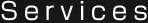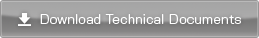## Motor Shaft Conversion Torque: TO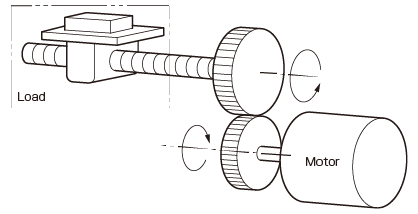Z1 : Teeth number of the motor-side gear
Z 2 : Teeth number of the load-side gear
R : Gear ratio Z2/Z1
η : Transmission efficiency % /100

 To= R･T [N･m] η

## Torque when Adding the External Force of the Rotating Object: TD : Diameter of the drum [m]
F : External force[N]

 T= 1 D･F [N･m] 2

## Torque by Friction Force of the Feed Screw and External Force: TM : Mass of the load [kg]
g : Gravitational acceleration[m/s2]
μ : Friction coefficient of the feed screw
F : External force[N]

 T= 1 P(F+μMg) [N･m] 2π

(Normally, μ : approximately 0.05 to 0.2)

## Torque by Friction Force of Rack and Pinion and External Force: T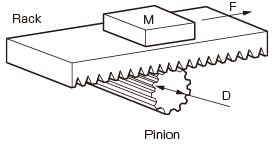D : Diameter of the pinion [m]
M : Mass of the load [kg]
g : Gravitational acceleration[m/s2]
μ : Friction coefficient of the rack and pinion
F : External force[N]

 T= 1 D(F+μMg)[N･m] 2

(Normally, μ : approximately 0.08 to 0.1)

## Torque by Friction Force of Conveyor and External Force: T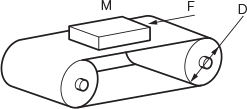D : Diameter of the roller [m]
M : Mass of the load [kg]
g : Gravitational acceleration[m/s2]
μ : Friction coefficient of the conveyor
F : External force [N]

 T= 1 D(F+μMg)[N･m] 2

(Normally, μ : approximately 0.05 to 0.1)

* The friction coefficient value μ varies depending on the machine processing accuracy, lubrication condition, and other factors.
Pay enough attention when calculating the load torque.
* The transmission efficiency η between the motor and load varies depending on the connection method. The following shows typical values.
Spur gear: 0.85　　Chain: 0.9　　V-belt: 0.9

Other Technical Documents

﻿